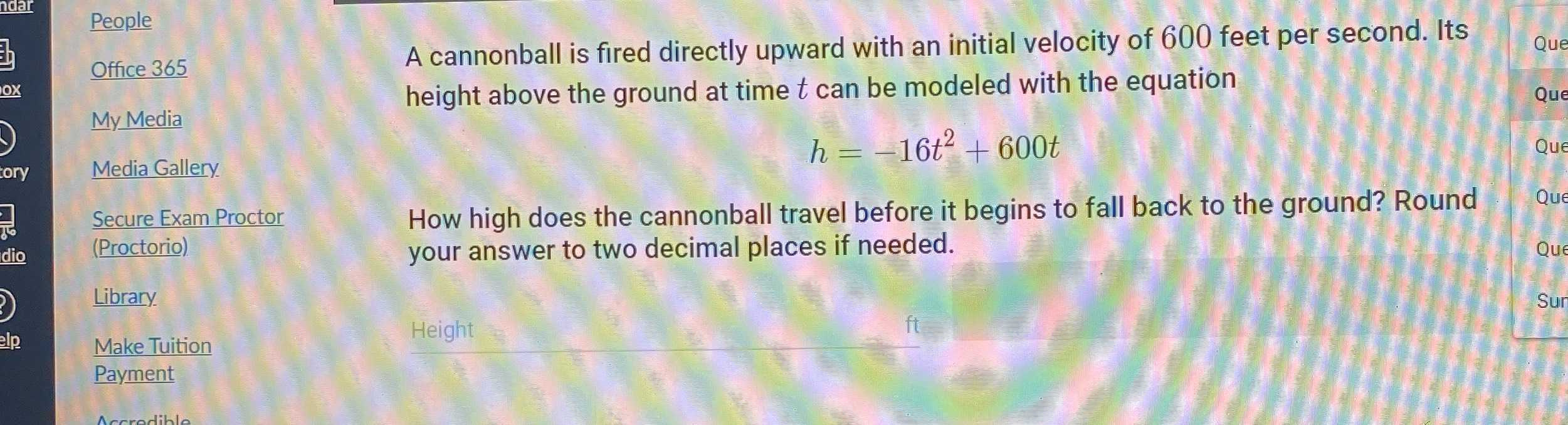### ¿Todavía tienes preguntas de matemáticas?

Pregunte a nuestros tutores expertos
Algebra
PreguntaA cannonball is fired directly upward with an initial velocity of $$600$$ feet per second. Its height above the ground at time $$t$$ can be modeled with the equation

$$h = - 16 t ^ { 2 } + 600 t$$

How high does the cannonball travel before it begins to fall back to the ground? Round your answer to two decimal places if needed.

$$h_{\max}= \frac{- 600^{2}}{4(- 16)}= 5625$$